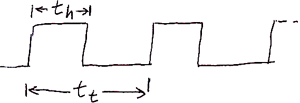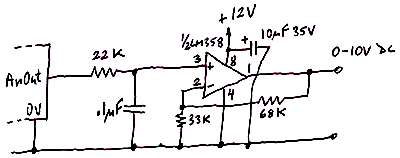# Understanding EC1 analog outputs

NOTICE: SPLat Controls has moved. We are now at 1/85 Brunel Rd, Seaford, 3198. map

In this EasyStep you get a quick overview of the standard analog outputs on the EC1

Expert summary

• The analog outputs actually generate a 3.3V pulse width modulated (PWM) pulse train;
• fAnOut instruction gives 0% to 100% output duty cycle for values from 0.0 to 1.0 in floating point register W
• Accuracy: 3% (unloaded voltage level)
• Resolution: Varies with base frequency, typically 15 to 16 bits;
• Output impedance: See Understanding EC1 digital outputs;
• The base frequency can be programmed, to suit varying requirements such as driving RC (hobby) servos.

Close

The pins on the EC1 designated as analog outputs generate a Pulse Width Modulated (PWM) stream of digital pulses (also called a pulse train). So you don't get an analog voltage directly, but with some very simple external circuitry you can convert the pulses into an analog voltage. This actually adds to the versatility of the EC1 without adding one cent to the price.

The sketch below shows what a PWM pulse stream looks like (time on the horizontal axis, voltage on the vertical axis. The total period (the time from the start of one pulse until the start of the next pulse is denoted by tt. The time the pulse is high (in our case at 3.3V) is denoted by th. The duty cycle is the percentage or fraction of the total period that the voltage is high. That can be calculated as

P = th / tt## Using the raw PWM signal

The raw pulse train can be used for things like driving an LED with variable brightness. While the LED may be flashing at a very high frequency, the eye will not discern the individual flashes. All the eye will see is the average light output, which is proportional to the duty cycle of the pulse stream. This EasyStep shows you how to do it with an RGB 3-colour LED.

In another EasyStep I will show you also how to control and RC (hobby) servo.

## A very simple conversion to an analog voltage

The simplest way to convert the PWM pulse train to a DC voltage is with an resistor-capacitor (RC) lowpass filter. What the filter does is to average the voltage coming out of the EC1. The sketch below is a schematic of an RC filter connected to an EC1.The voltage coming out of the filter will not be a perfectly steady voltage. It will fluctuate slightly at the frequency of the PWM signal. These fluctuations are called ripple. Ripple can never be eliminated, but it can be reduced to a point where it doesn't matter.

A RC lowpass filter is characterised by its time constant. To calculate the time constant, simply multiply the resistor value in ohms by the capacitor value in farads. Resistors typically come in values of thousands of ohms (kilo-ohms or kΩ or just K). Capacitors typically come in values of millionths of a farad (a farad is a LOT!) (micro-farad or μF). The larger the time constant of the filter, more effective it will be at reducing the PWM ripple. The downside is that a large time constant will also slow down the filter's ability to change the output voltage quickly when your program changes the PWM duty cycle (by the way, we often just say "the PWM is 25%" when we actually mean the duty cycle is 25%).

Generally a time constant of 10 to 50 times the period of the PWM signal (tt above) is suitable.

Example: The default PWM frequency in the EC1 is 40kHz. This gives a period of 1/40kHz = 25μS. In the circuit above, if the resistor R is 10kΩ and the capacitor is 0.1μF, the time constant would be

τ = 10000 * 0.01E-6 = 0.001S = 1mS

Which is 40x the PWM period.

The downside of this simple technique is that the resistor gives the circuit a high output resistance. That means that anything resistive connected to the output of the filter will "drag down" the voltage. For example, if the filter resistor is 10kΩ, a load of 100;Ω will pull it down by about 10%.

## Amplifying to 10V DC

The sketch below shows teh schematic of a circuit that will filter the PWM pulse train and amplify it up to a 0 to 10V analog output with a low output resistance. This circuit can drive load resistances as low as 2kΩ (5mA load current). It is the circuit used in out industrial grade off the shelf controllers.The amplifier chip is an LM358. This contains two identical operational amplifiers, of which this circuit only uses one. I selected 22K for the filter resistor because that gives the best DC accuracy. The resultant filter time constant is 2.2mS. The output reistance of this circuit will be very low.

## Resolution

The resolution of the analog/PWM outputs is 10 bits, or 0.1%.

## Accuracy

While the resolution is quite high, the accuracy is not. See Analog Accuracy is expensive, Resolution is cheap. The main governing factor of accuracy of any digital to analog converter is the accuracy of the reference voltage. In the EC1 the reference voltage is the 3.3V supply, which comes from a MCP1801 3.3V regulator with a worst case accuracy of 2.5% (it is typically better than that, but professionals use worst case numbers!). If you have a good digital multimeter, you can get a "fix" on the actual reference voltage by measuring between a 0V pin and a 3.3V pin.

If you need more accuracy you will have to somehow calibrate your system.

## Some interesting ways to blow up your EC1

If you want to blow up your EC1, and void the warranty, here are some things you can do to the analog outputs:

• Connect an analog output to any voltage greater than 3.3V;
• Connect an analog output to any voltage more negative than 0V;